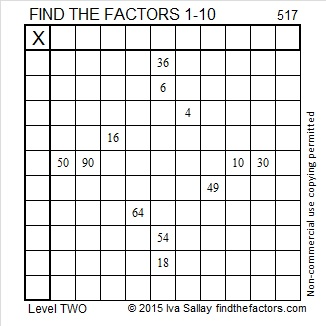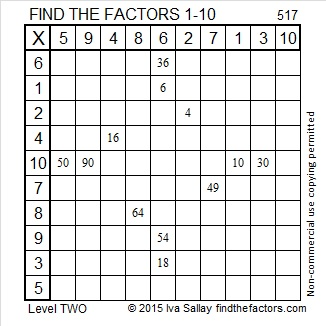# 517 and Level 2

5 – 1 + 7 = 11 so 517 can be evenly divided by 11.

517 = 97 + 101 + 103 + 107 + 109 which is all the prime numbers between 90 and 112.Print the puzzles or type the solution on this excel file: 10 Factors 2015-06-08

—————————————————————————————————

• 517 is a composite number.
• Prime factorization: 517 = 11 x 47
• The exponents in the prime factorization are 1 and 1. Adding one to each and multiplying we get (1 + 1)(1 + 1) = 2 x 2 = 4. Therefore 517 has exactly 4 factors.
• Factors of 517: 1, 11, 47, 517
• Factor pairs: 517 = 1 x 517 or 11 x 47
• 517 has no square factors that allow its square root to be simplified. √517 ≈ 22.737634—————————————————————————————————